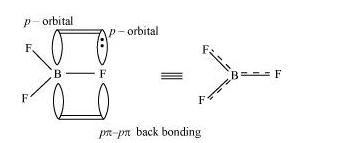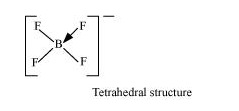# Suggest reasons why the B–F bond lengths in

Question:

Suggest reasons why the B-F bond lengths in $\mathrm{BF}_{3}(130 \mathrm{pm})$ and $\mathrm{BF}_{4}^{-}(143 \mathrm{pm})$ differ.

Solution:

The $\mathrm{B}-\mathrm{F}$ bond length in $\mathrm{BF}_{3}$ is shorter than the $\mathrm{B}-\mathrm{F}$ bond length in $\mathrm{BF}_{4}^{-} . \mathrm{BF}_{3}$ is an electron-deficient species. With a vacant $p$-orbital on boron, the fluorine and boron atoms undergo $p \pi-p \pi$ back-bonding to remove this deficiency. This imparts a double-bond character to the B-F bond.This double-bond character causes the bond length to shorten in $\mathrm{BF}_{3}(130 \mathrm{pm})$. However, when $\mathrm{BF}_{3}$ coordinates with the fluoride ion, a change in hybridisation from sp $^{2}$ (in $\mathrm{BF}_{3}$ ) to $s p^{3}$ (in $\mathrm{BF}_{4}^{-}$) occurs. Boron now forms $4 \sigma$ bonds and the double-bond character is lost. This accounts for a B-F bond length of 143 pm in $\mathrm{BF}_{4}^{-}$ion.